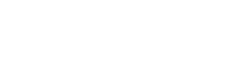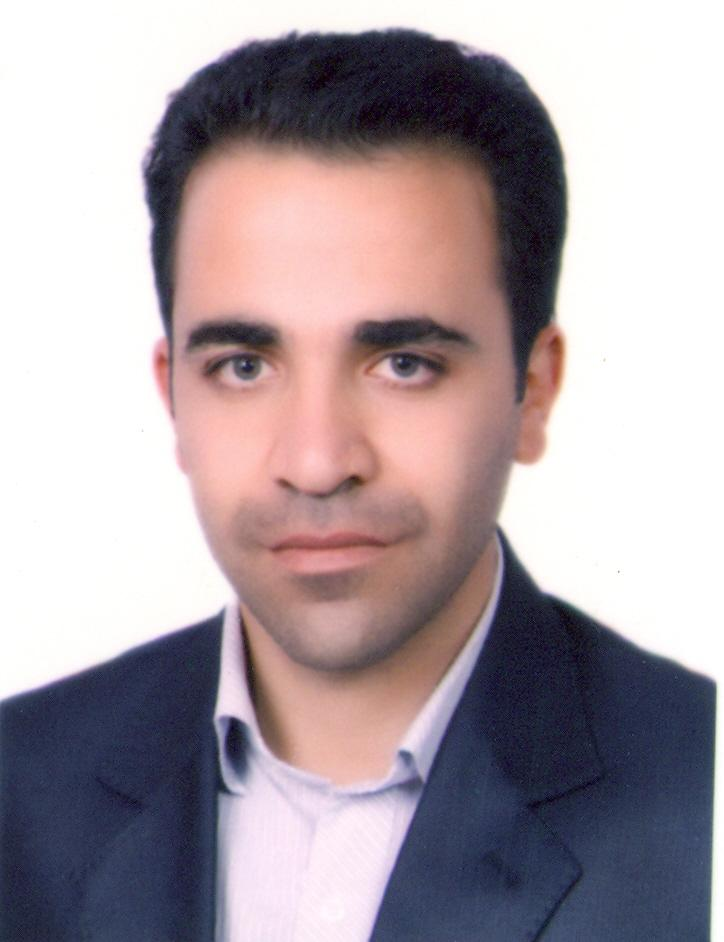سه شنبه ٥ مهر ١٤٠١محمد رضا احمدی دارانی

استادیار

علوم کامپیوتر

دانشکده علوم ریاضی

تلفن: 03832324401

##### مقالات
###### International Journal
1. A Ansari, M.A. Darani, New Inversion Techniques for Some Integral Transforms via the Generalized Product Theorem of the Mellin Transform , ISRN Applied Mathematics 2012
2. M.A. Darani, S. Bagheri, Fractional type of flatlet oblique multiwavelet for solving fractional differential and integro-differential equations , Computational Methods for Differential Equations 2 (4), 268-282
3. M.A, Darani, M. Nasiri, A collocation method for solving the fractional calculus of variation problems , Boletim da Sociedade Paranaense de Matemática 35 (1), 163-172
4. M.H. Derakhshan, A. Ansari, M.A. Darani, On asymptotic stability of Weber fractional differential systems , Computational Methods for Differential Equations 6 (1), 30-39
5. M.A. Darani, H. Adibi, M. Lakestani, Numerical solution of nonlinear ordinary differential equations using flatlet oblique multiwavelets , International Journal of Computer Mathematics 88 (5), 1035-1051
6. A Ansari, M Ahmadi Darani, On the generalized mass transfer with a chemical reaction: Fractional derivative model , Iranian Journal of Mathematical Chemistry 7 (1), 77-88
7. M. Ahmadi, H. Adibi, The Chebyshev tau technique for the solution of Laplace’s equation , Applied mathematics and computation 184 (2), 895-900
8. M.H. Derakhshan, M.A. Darani, A Ansari, R Khoshsiar Ghaziani, On asymptotic stability of Prabhakar fractional differential systems , Computational Methods for Differential Equations
9. M.R. Darani, Direct meshless local Petrov Galerkin method for the two- dimensional Klein-Gordon equation , Engineering Analysis with Boundary Elements
10. M.A. Darani, H. Adibi, M. Lakestani, Numerical solution of integro-differential equations using flatlet oblique multiwavelets , Dynamics of Continuous, Discrete & Impulsive Systems. Series A
11. M.R. Darani, H. Adibi, R.P Agarwal, R Saadati, Flatlet oblique multiwavelet for solving integro-differential equations , Dynamics of Continuous, Discrete and Impulsive Systems, Series A
12. Mohammadreza Ahmadi Darani, Mitra Nasiri, A fractional type of the Chebyshev polynomials for approximation of solution of linear fractional differential equations , A fractional type of the Chebyshev polynomials for approximation of solution of linear fractional differential equations M Ahmadi Darani, M Nasiri Computational Methods for Differential Equations 1 (2), 96-107
13. A. Ansari, M.A. Darani, M. Moradi, On Fractional Mittag–Leffler Operators , ON FRACTIONAL MITTAG–LEFFLER OPERATORS, REPORTS ON MATHEMATICAL PHYSICS 70:119-131
14. Mohammadreza Ahmadi Darani, Abbas Saadatmandi, The operational matrix of fractional derivative of the fractional-order Chebyshev functions and its applications ,
###### International Conference
15. M.A. Darani, A. Ansari, Numerical solution of nonlinear ordinary differential equations using Bernstein polynomials and their orthonormal duals , The 43 th Annual Iranian Mathematics Conference, 2013.
16. M.H. Derakhshan, M.A. Darani, A numerical scheme for two-dimensional optimal control problems with Grünwald-Letnikov for Riesz Fractional Derivatives , The 46 th Annual Iranian Mathematics Conference, 542, 2015
17. M.R. Ahmadi, G. Banimahdi, New rational approximation of Mittag-Leffler function with orthogonal polynomials , The 46 th Annual Iranian Mathematics Conference, 319, 2015
###### National Conference
18. N.C.K. Abad, M.A. Darani, An approximation of a two-dimensional Volterra-Fredholm integral equations via Inverse Multiquadric RBFs , The 46 th Annual Iranian Mathematics Conference, 790, 2015

##### افتخارات

کلیه حقوق سایت برای دانشگاه شهرکرد محفوظ است